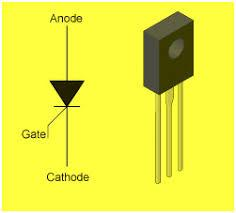# Basic Power Electronics (22427) Quiz

25 Questions | Total Attempts: 68SettingsCreate your own QuizMr. Gavde P. B. M. E. (Electronics) (Lecturer in Electronics) Electronics & communication Department Government Polytechnic,Beed

• 1.
Latching current define as ........
• A.

Minimum on state current required to keep the device in the on state after the triggering pulse has been removed SCR will not Turn off

• B.

Minimum on state current required to keep the device in the on state after the triggering pulse has been removed SCR will remain Turn ON

• C.

Minimum on state current required to keep the device in the on state after the triggering pulse has been removed SCR will  Turn off

• D.

Both 1 & 2

• 2.
Holding current define as .......
• A.

It is mimimum forward anode current required to keep SCR on if the current fall below the holding level,then SCR remain in  turn on

• B.

It is mimimum forward anode current required to keep SCR on if the current fall below the holding level,then SCR   turn off

• C.

Both 1 &  2

• D.

None of above

• 3.
I=    α2Ig+IcEo   /   (1-α)   in this equation if α approaches to unity then anode current will be
• A.

Zero

• B.

Minimum

• C.

Increases

• D.

None of above

• 4.
If value of latching current is 12mA what is the value of holding current.....
• A.

10mA

• B.

13mA

• C.

14mA

• D.

15mA

• 5.
For 4000V,3000A GTO need .........gate current to Turn Off
• A.

750A

• B.

-750A

• C.

780A

• D.

-780A

• 6.
In TRIAC which mode is less sensitive
• A.

Mode I & II

• B.

Mode II & III

• C.

Mode I & IV

• D.

Mode III & IV

• 7.
Arrow in the MOSFET symbol indicates..........
• A.

Direction of Hole flow

• B.

Dirction of Current flow

• C.

Direction of electron flow

• D.

All of the above

• 8.
Power MOSFET is .....
• A.

Voltage control Device

• B.

Current control Device

• C.

Both 1 & 2

• D.

None of above

• 9.
In IGBT three terminals are .....
• A.

Drain ,source & Gate

• B.

Collector,Emitter & Gate

• C.

Drain,Source& Emitter

• D.

Both 1 & 2

• 10.
In which condition IGBT Turn on
• A.

VGS>VGS(TH)

• B.

VGS

• C.

VGS=VGS(TH)

• D.

VGS=0

• 11.
Interbase resistance (RBB) in UJT exist between.....
• A.

3K Ohm to 4K Ohm

• B.

1K Ohm to 3k Ohm

• C.

4K Ohm to 10k Ohm

• D.

10K Ohm to 14k Ohm

• 12.
Intrinsic stand off ratio range in between .....
• A.

0.2 to 0.4

• B.

0.3 to 0.5

• C.

0.9 to 1.3

• D.

0.5 to 0.8

• 13.
Which device is called as uncontrolled rectifier
• A.

SCR

• B.

Diode

• C.

BJT

• D.

PUT

• 14.
In 2 quadrant converter polarity of output voltage can be either  positive of negative however output current has one polarity only called as.......
• A.

Full converter

• B.

Semiconverter

• C.

Dual Converter

• D.

Both 1& 2

• 15.
Which one is not function of freewheeling diode......
• A.

It prevent reversal of load voltage except for small voltage drop

• B.

It convert AC to pulsating DC

• C.

It transfer the load current away from the main rectifier,thereby allowing all of its thyristor to regain their blocking state

• D.

None of above

• 16.
In three phase half wave controlled rectifier with resistive load primary is.....
• A.

Star connection

• B.

Transformer connection

• C.

Delta connection

• D.

Both 1 & 3

• 17.
In 3 phase half wave controlled rectifier conduction period for each thyristor is equal to ....
• A.

60 degree

• B.

80 degree

• C.

100 degree

• D.

120 degree

• 18.
If dc circuit of 240V is to be protected with 25V selenium cell then how many cells required
• A.

10

• B.

12

• C.

14

• D.

16

• 19.
For Thyristor overcurrent protection which is better option
• A.

Selenium diode protection

• B.

Snubber circuit protection

• C.

Crowbar protection

• D.

Metal oxide varistor

• 20.
How to turn off SCS
• A.

Reduce anode current below IH

• B.

By applying negative gate pulse to GK

• C.

By applying positive gate pulse to GA

• D.

All of the above

• 21.
Which device is complementry SCR
• A.

SUS

• B.

SBS

• C.

LASCR

• D.

PUT

• 22.
Which method is more reliable  & efficient to turn ON SCR
• A.

Forward voltage triggering

• B.

Gate triggering

• C.

Dv/dt triggering

• D.

Thermal triggering

• 23.
Reverse characteristics of SCR similer to the...
• A.

TRIAC

• B.

DIAC

• C.

Diode

• D.

UJT

• 24.
Device used for a chopper can be represented by
• A.

Circle

• B.

Square block

• C.

Switch with an arrow

• D.

Zig-zac

• 25.
In light Dimmer if Pot is in mid position then intensity of light is
• A.

Medium intensity

• B.

Low intensity

• C.

High intensity

• D.

Bulb in off condition

Related TopicsBack to top
×

Wait!
Here's an interesting quiz for you.